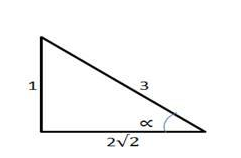# The length of the perpendicular segment from the origin`
Question:

The length of the perpendicular segment from the origin to a line is 2 units and the inclination of this perpendicular is ∝ such that sin $\propto=\frac{1}{3}$ and ∝ is acute Find the equation of the line.

Solution:

To Find: The equation of the line .

Given : $\mathrm{p}=2$ units and $\sin \propto=\frac{1}{3}$.

Since $\sin \propto=\frac{\text { opp }}{\text { hyp }}=\frac{1}{3}$Using Pythagoras theorem:

$\operatorname{adj}=\sqrt{9-1}=\sqrt{8}=2 \sqrt{2}$ units.

i.e; $\cos \alpha=\frac{\text { adj }}{\text { hyp }}=\frac{2 \sqrt{2}}{3}$

Formula used:

equation of the line: $x \cos \alpha+y \sin \alpha=p$

$x \times\left(\frac{2 \sqrt{2}}{3}\right)+y \times\left(\frac{1}{3}\right)=2$

Hence, $2 \sqrt{2} x+y=6$ Or $\sqrt{8} x+y=6$ is the required equation of the line.